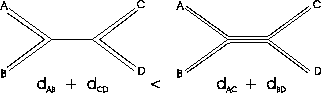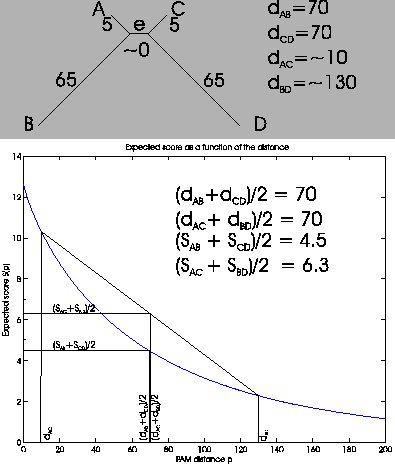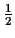Next: How to Make Scores Up: Dayhoff Scores and Evolutionary Previous: Monotonicity of scores and

## Scores are inconsistent to build phylogenetic trees

Imagine a tree as in Figure 3. In this tree, the PAM distances follow

 dAB + dCD < dAC + dBD (4)

If scores were consistent to build trees, then the sum of the first two scores must be larger than the sum of the other two scores (see Figure 3):

 SAB + SCD > SAC + SBD (5)As we have seen, the expected score SD(d) as a function of d is not a straight line. This means that the scores are not consistent. We now show this with a simple geometrical proof.

Theorem 2.1   dAB + dCD < dAC + dBD does not imply SAB + SCD > SAC + SBDProof 2.1   To prove this, we construct a tree with four leaves, where two edges are very long and the other edges are very short (see Figure 4 on top). We choose the length of the middle edge e to be close to zero. We also choose the distances so that dAB + dCD = dAC + dBD. For simplicity we look atof the distances and scores. As you can see in Figure 4, both sums of distances are the same: (dAB + dCD)/2 = 70, and (dAC + dBD)/2 = 70. The scores can be read from the graph. The score (dAC + dBD)/2 is the midpoint of the line between SAC and SBD. The intersection with the y axis gives us the score, which is about 6.3. We do the same for (SAB + SCD)/2. This case is simpler, as both values are the same, so we already have the midpoint. The score is around 4.5. If the graph is not a straight line, there will be points where the sum of the distances are the same, and sum of the scores are different. Now we change the length of edge e slightly by adding a very small amount. (dAC + dBD)/2 is now slightly greater than 70, but the score (SAC + SBD)/2 is still clearly larger than the score (SAB + SCD)/2. Even though dAB + dCD < dAC + dBD, the condition for the scores, SAB + SCD > SAC + SBD, does not hold. If the curvature was negative, then we would move (dAC + dBD)/2 to be slightly lower than 70. Unless there is no curvature, i.e. S(d) is a straight line, we can always find a counter example.

The conclusion is, if we use scores derived from Dayhoff matrices to construct trees, we could obtain incorrect results, no matter how much data is available. In the example, we would decide to connect leaves AC and BD, not AB and CD. Felsenstein  noted a similar result for parsimony. This means that scores derived from Dayhoff matrices should not be used to construct evolutionary trees. They are positively misleading in some cases as is parsimony.
The result is more general. Whatever scoring matrix E we use, if the expected score SE(d) as a function of d is not a straight line, we can derive counterexamples like the one in Figure 4. As SE(d) is a linear combination of exponentials in d (equation 2), it will never be a straight line. So no scoring matrix E can give consistent scores usable for constructing trees.Next: How to Make Scores Up: Dayhoff Scores and Evolutionary Previous: Monotonicity of scores and
Chantal Korostensky
1999-07-14